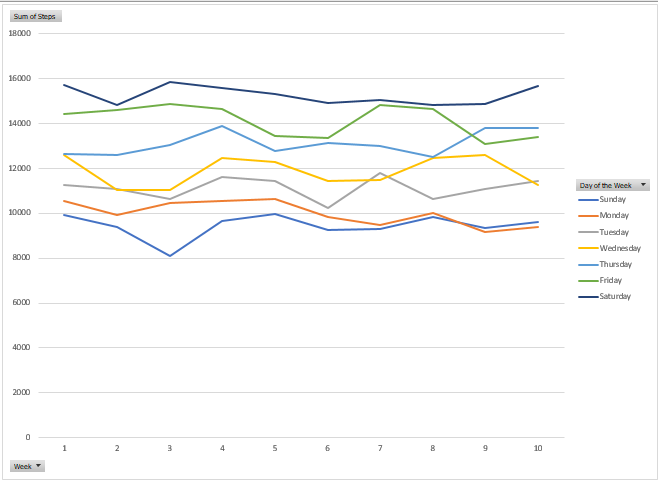# Excel Date and Time Functions

Another installment of our educational blog series is here! If you have suggestions for future blog topics, we want to hear them. Email us at marketing@capitalizeconsulting.com with your ideas!

Excel has numerous date and time functions that are very powerful in manipulating and deriving dates and times. In these examples, we are going to use one of them – WEEKDAY – as well as supporting numerical functions – FLOOR and TEXT.

In this first example, I have a goal of walking an average of 10,000 steps a day for the next 10 weeks, and would like to track my progress via a scorecard. I have a spreadsheet where I will log my steps daily and need to report weekly whether my average step count meets my goal of 10,000 steps.

I have built a simple data tracker to track my daily steps: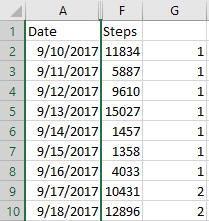My next step is to be able to report weekly to see if I am meeting my goal. To do so, I will use the date function DAYS, along with supporting function FLOOR, in order to derive the week number (1, 2, 3, … 10). I want to determine what week each date falls into, relative to my start date of 9/10/2017. Looking at the data above, 9/10 – 9/16 would fall into Week 1, 9/17 – 9/23 into Week 2, and so on, but I want Excel to determine the week number for me.

I start by determining the number of days that have elapsed since the start of my goal, using the DAYS function. The DAYS function takes two arguments – end date and start date – and returns the number of days between those two dates. For end date, I pass the date cell for that row, and for start date, I pass an absolute reference of the start date of my goal (9/10/2017) in this case. As shown in the below screenshot, I have now calculated the Days from the Start using the formula “=DAYS(A2,\$A\$2)”, entered once, and dragged to the other cells: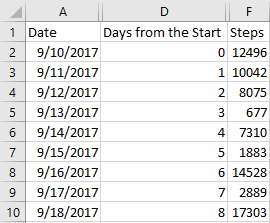Now that I have Days from the Start, I can derive the week that each date falls in using the FLOOR function. In our example, those dates less than 7 days from the start are in Week 1, greater than 7 but less than 14 days are in Week 2, and so on. In other words, if we ignore the remainder, always rounding down, then the formula of (Days from the Start / 7 + 1) would give you the Week. The FLOOR function does just that. It takes whatever number is given and rounds that down to the nearest specified multiple. FLOOR takes two arguments – number and multiple. The function then rounds the number down to the nearest multiple passed.

See below for a few examples of the result FLOOR would output, given the inputs of number and multiple specified: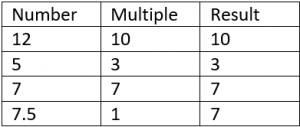In our case, we need to derive the week from the given date, knowing we have a start date of 9/10. We can do that using FLOOR, one of two ways.

1. “=FLOOR(D2/7,1)+1” – Take the number of days and divide by 7 to derive the fraction of week “completed,” adding 1 so that we start with week 1 rather than week 0.
2. “=FLOOR(D2,7)/7+1” – Take the number of days and round to the nearest multiple of 7 (0, 7, 14, etc.). Divide that result by 7 to convert to Week, and again add 1 so that we start with week 1 rather than week 2.

Either formula will produce the same result, shown below: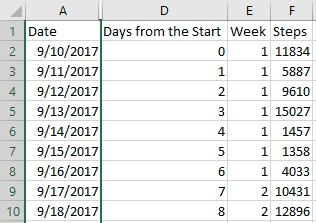Armed with the derived Week, we can now create a quick Pivot Table showing me the average steps per week: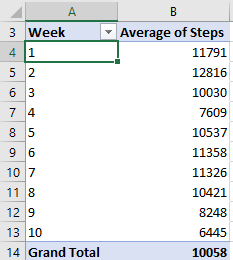Now that I have my average steps by week, I would like to dive in deeper to see if there are any trends in my walking pattern by day of the week. For example, do I walk more on the weekends when I am not as busy with work?

To glean any patterns associated with day of the week, I first need to determine each day of the week. To do so, I can use the WEEKDAY function. The WEEKDAY function returns the day of the week, in numeric value, of a date passed. The function has two parameters – the date passed, which is required, and an optional parameter called “return type” which determines what days the numbers 1 through 7 correspond to. By default, a return type of 1 is used, meaning a value of 1 is Sunday and a value of 7 is Saturday. If you want to use the default value, you do not need to enter an argument for return type. See below for the full list of return types: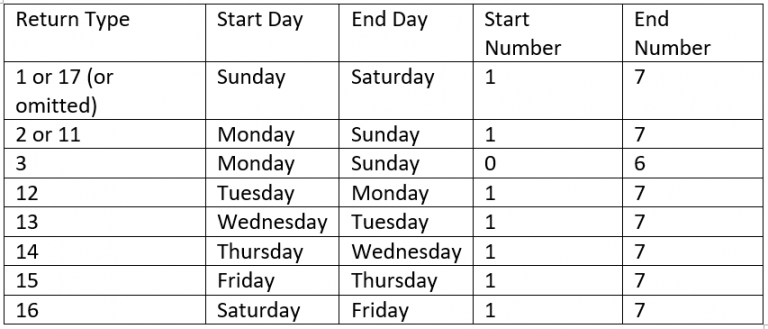Excel prompts you for return type when entering a new formula, so you won’t need to memorize the return types associated with each configuration: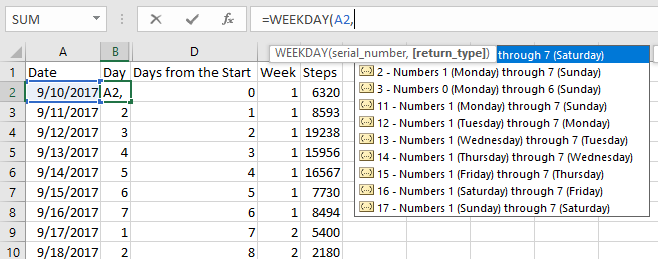In my case, the default return type was all I needed, so my formula read, “=WEEKDAY(A2)”, with results shown below: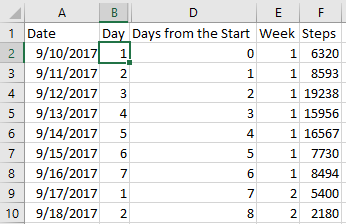While I now have what I need to report steps by day of the week, I wanted my report to actually show day of the week, rather than a number. To do so, I used the TEXT function. The TEXT function is used to convert a numeric or date value into text, based on certain criteria. The function takes two arguments – the value to be converted, and a format text parameter which defines the formatting criteria to be used. The format text parameter, in the context of date, has the following definitions: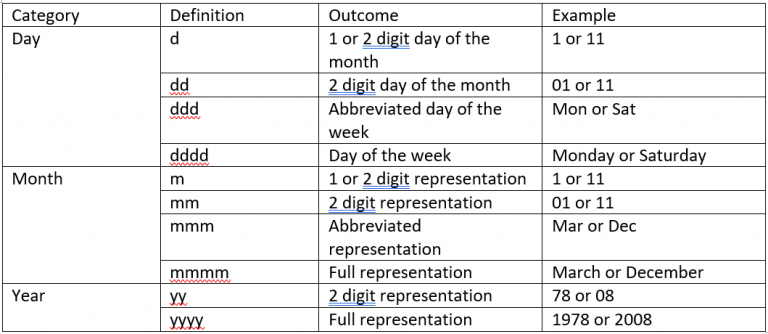Using the TEXT function, I am able to derive the day of the week with the following formula: “=TEXT(B2,”dddd”)”. Or, if we wanted to derive in one step, we could via the formula: “=TEXT(WEEKDAY(A2),”dddd”)”. Final data for use in our analysis is: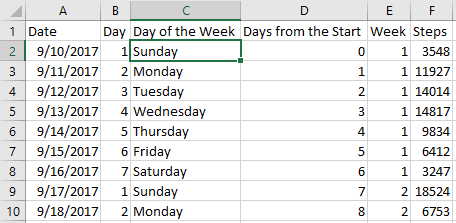Now that we have our steps by day of the week, we can move forward with analyzing our daily patterns. There are many examples to build, but I have chosen two.

1. A pivot table that shows my average weekly step count, with ability to drill down to see steps by day of the week: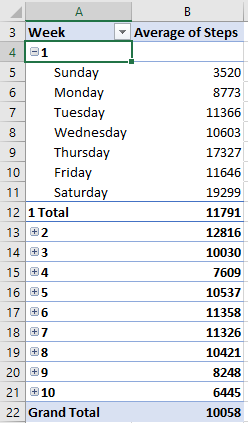2. A chart that shows, by day, steps by week